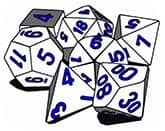Mystery
Numerology

# Numerology Master NumbersMaster numbers are incredibly important in the Numerology. The association of numerology to divination and occult in today's world is known to everyone. First developed by a renowned mathematician Pythagoras in 600 B.C., numerology is becoming popular parallel to astrology.

Every alphabet is given a particular number in numerology and the names of people correspond to various single digit numbers, for example: Mary Smith, the corresponding value of the alphabets is given in the Pythagorean table. Name number: 4+1+9+7+1+4+9+2+8 = 45. Reduce the above value to a single digit by adding them, 4 + 5 = 9. Similarly, DOB= 28-04-1968

Birth number: 2+8+0+4+1+9+6+8 = 38; 3 + 8 = 11. This is again reduced to single digit, 1 + 1 = 2. The birth number is also known as life path number. Now the names are reduced to single digits or single vibrant numbers by adding the last double digit value but there are a few double digit numbers that are special and are considered to be the most vibrant. These are eleven (11), twenty-two (22), thirty-three (33) and forty-four (44). These numbers are considered under a different category altogether and are called as Master Numbers. According to various numerologists from http://numerology.center, the master numbers are very challenging and it takes time to integrate these vibrant numbers into one's personality traits. These numbers emphasis on a greater learning and experiences and possess strong vibration. This is one reason why master numbers are known as late bloomers.

Master numbers are considered very strong and have the qualities of the digit they add up to. For example, the master number eleven (11) adds up to 2, (1+1=2) therefore it has all the traits of the number 2. The Master number forty four (44) is considered to be the strongest among the four, as a person born under its influence can create a magic around him and others.